TU Vienna logic.at Theory and Logic Group (E185-2)

## Projection and Refutation

Every clause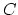in the characteristic clause set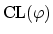of a skolemized proof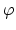defines a proof projection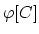.is defined fromby omitting all inferences on ancestors of cuts, leaving the clauseas a residue'' in the end-sequent. Equality and definition rules appearing within the input proof are propagated to the projections like any other rules. In fact,is a cut-free proof of the end-sequent augmented by. For a formal definition of projection we refer to  and .

The construction ofis illustrated below.

Example 4   Letbe the proof of the sequent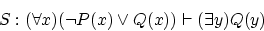as defined in Example 3. We have shown that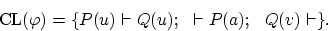We now define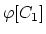, the projection ofto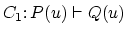:
The problem can be reduced to a projection in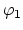because the last inference inis a cut and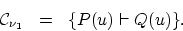By skipping all inferences inleading to the cut formulas we obtain the deduction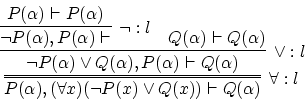In order to obtain the end-sequent we only need an additional weakening and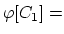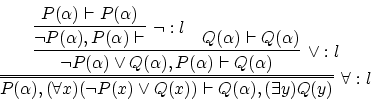For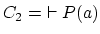we obtain the projection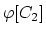: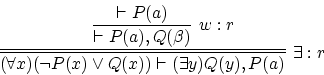Similarly we obtain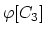: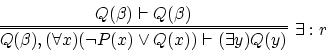We have seen that, in the projections, only inferences on non-ancestors of cuts are performed. If the auxiliary formulas of a binary rule are ancestors of cuts we have to apply weakening in order to obtain the required formulas from the second premise.

Letbe a proof of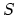s.t.is skolemized and let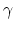be a ground resolution refutation of the (unsatisfiable) set of clauses. Thencan be transformed into a proof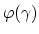ofwith at most atomic cuts.is constructed fromsimply by replacing the clauses by the corresponding proof projections. The construction ofis the essential part of the method CERES (the final elimination of atomic cuts -- provided it is possible at all -- is inessential). The resolution refutationcan be considered as the characteristic part ofrepresenting the reduction to atomic cuts. Below we give an example of a construction of.

Example 5   Letbe the proof of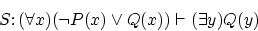as defined in Examples 3 and 4. Then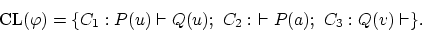First we define a resolution refutation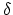of: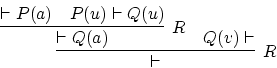and a corresponding ground refutation: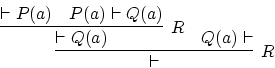The ground substitution defining the ground projection is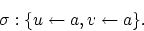Let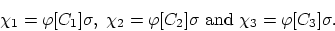Moreover let us write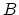for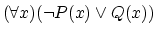andfor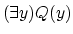.

Thenis of the form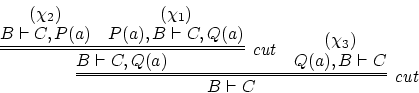Finally we give the general definition of CERES (cut-elimination by resolution) as a whole. As an input we take skolemized proofs.

• construct,
• construct a resolution refutationof,
• compute the output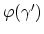for a ground projection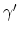of.

Theorem 2   CERES is a cut-elimination method, i.e., for every skolemized proofof a sequent, CERES produces a proof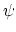ofs.t.contains at most atomic cuts.